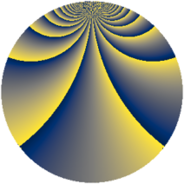# Properties

 Label 1344.4.ccLevel $1344$ Weight $4$ Character orbit 1344.cc Rep. character $\chi_{1344}(431,\cdot)$ Character field $\Q(\zeta_{12})$ Dimension $752$ Sturm bound $1024$

# Related objects

## Defining parameters

 Level: $$N$$ $$=$$ $$1344 = 2^{6} \cdot 3 \cdot 7$$ Weight: $$k$$ $$=$$ $$4$$ Character orbit: $$[\chi]$$ $$=$$ 1344.cc (of order $$12$$ and degree $$4$$) Character conductor: $$\operatorname{cond}(\chi)$$ $$=$$ $$336$$ Character field: $$\Q(\zeta_{12})$$ Sturm bound: $$1024$$

## Dimensions

The following table gives the dimensions of various subspaces of $$M_{4}(1344, [\chi])$$.

Total New Old
Modular forms 3136 784 2352
Cusp forms 3008 752 2256
Eisenstein series 128 32 96

## Trace form

 $$752q + 2q^{3} + 16q^{7} + O(q^{10})$$ $$752q + 2q^{3} + 16q^{7} - 16q^{13} + 4q^{19} + 50q^{21} + 8q^{27} - 4q^{33} - 4q^{37} + 4q^{39} + 16q^{43} + 498q^{45} - 16q^{49} + 810q^{51} + 32q^{55} - 4q^{61} - 1628q^{67} - 116q^{69} + 360q^{75} - 4q^{81} - 1016q^{85} + 4q^{87} + 4552q^{91} - 110q^{93} - 32q^{97} - 2908q^{99} + O(q^{100})$$

## Decomposition of $$S_{4}^{\mathrm{new}}(1344, [\chi])$$ into newform subspaces

The newforms in this space have not yet been added to the LMFDB.

## Decomposition of $$S_{4}^{\mathrm{old}}(1344, [\chi])$$ into lower level spaces

$$S_{4}^{\mathrm{old}}(1344, [\chi]) \cong$$ $$S_{4}^{\mathrm{new}}(336, [\chi])$$$$^{\oplus 3}$$